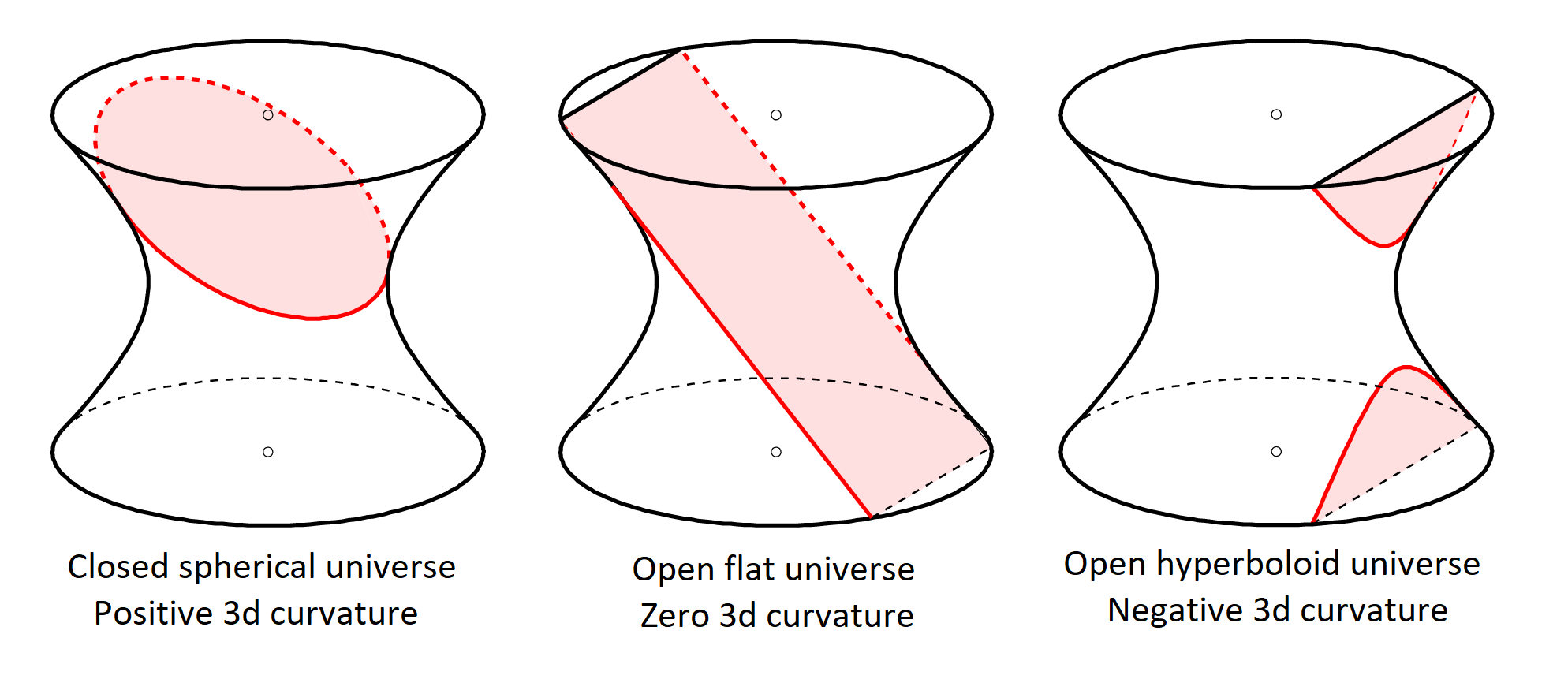# nLab de Sitter spacetime

Contents

### Context

#### Riemannian geometry

Riemannian geometry

# Contents

## Definition

Full de Sitter spacetime is the Lorentzian manifold which is the coset space of Lorentz groups

$dS^d \simeq O(d,1)/O(d-1,1)$

This models a cosmology FRW model for positive cosmological constant/dark energy.

Similarly anti de Sitter spacetime is

$adS^d \simeq O(d-1,2)/O(d-1,1)$

(e.g here)

This admits different spatial slicings of relevance:graphics grabbed from this Physics.SE comment

geometric contextgauge groupstabilizer subgrouplocal model spacelocal geometryglobal geometrydifferential cohomologyfirst order formulation of gravity
differential geometryLie group/algebraic group $G$subgroup (monomorphism) $H \hookrightarrow G$quotient (“coset space”) $G/H$Klein geometryCartan geometryCartan connection
examplesEuclidean group $Iso(d)$rotation group $O(d)$Cartesian space $\mathbb{R}^d$Euclidean geometryRiemannian geometryaffine connectionEuclidean gravity
Poincaré group $Iso(d-1,1)$Lorentz group $O(d-1,1)$Minkowski spacetime $\mathbb{R}^{d-1,1}$Lorentzian geometrypseudo-Riemannian geometryspin connectionEinstein gravity
anti de Sitter group $O(d-1,2)$$O(d-1,1)$anti de Sitter spacetime $AdS^d$AdS gravity
de Sitter group $O(d,1)$$O(d-1,1)$de Sitter spacetime $dS^d$deSitter gravity
linear algebraic groupparabolic subgroup/Borel subgroupflag varietyparabolic geometry
conformal group $O(d,t+1)$conformal parabolic subgroupMöbius space $S^{d,t}$conformal geometryconformal connectionconformal gravity
supergeometrysuper Lie group $G$subgroup (monomorphism) $H \hookrightarrow G$quotient (“coset space”) $G/H$super Klein geometrysuper Cartan geometryCartan superconnection
examplessuper Poincaré groupspin groupsuper Minkowski spacetime $\mathbb{R}^{d-1,1\vert N}$Lorentzian supergeometrysupergeometrysuperconnectionsupergravity
super anti de Sitter groupsuper anti de Sitter spacetime
higher differential geometrysmooth 2-group $G$2-monomorphism $H \to G$homotopy quotient $G//H$Klein 2-geometryCartan 2-geometry
cohesive ∞-group∞-monomorphism (i.e. any homomorphism) $H \to G$homotopy quotient $G//H$ of ∞-actionhigher Klein geometryhigher Cartan geometryhigher Cartan connection
examplesextended super Minkowski spacetimeextended supergeometryhigher supergravity: type II, heterotic, 11d

### In perturbative quantum gravity

Discussion of de Sitter spacetime in perturbative quantum gravity and its infrared instability, includes the following:

### In string/M-theory

A no-go theorem for KK-compactifications of supergravity down to de Sitter spacetime is claimed in

• Juan Maldacena, Carlos Nunez, Supergravity description of field theories on curved manifolds and a no go theorem, Int.J.Mod.Phys. A16 (2001) 822-855 (arXiv:hep-th/0007018)

Discussion of de Sitter perturbative string theory vacua and their role in the landscape of string theory vacua or else in the swampland:

In view of alpha' higher curvature corrections:

• Ivano Basile, Alessia Platania, String Tension between de Sitter vacua and Curvature Corrections (arXiv:2103.06276)

“We show that the resulting higher-derivative $\alpha'$-corrections forbid the existence of de Sitter vacua, at least in regimes where string-loop corrections can be neglected.”

On de Sitter spacetime cosmology realized in brane world models in ambient $\sim$AdS-bulk spacetime:

Discussion in the context of M-theory on G2-manifolds:

For analogous discussion of embedding of the ekpyrotic cosmology-scenario into string theory/type IIA supergravity: